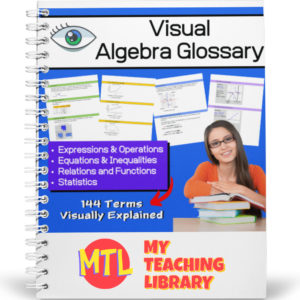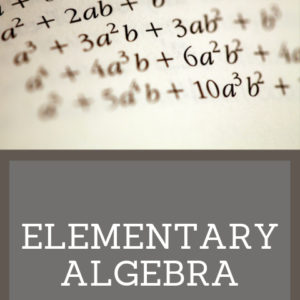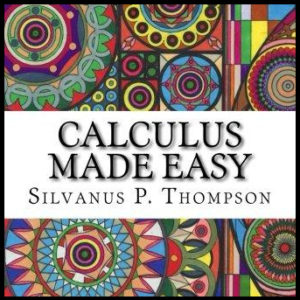Showing all 15 results

•\$3.00

Give your students the practice they need with the First Grade Math Skills Workbook. This fun, November themed review resource will give students extra practice in the following areas:

• – Addition facts to 20
• – Addition higher than 20 (no carry)
• – Finding the missing addends
• – Adding doubles to 24
• – Subtraction facts to 20
• – Word problems (Solving and explain through pictures) – addition and subtraction
• – Skip counting by 2 (Filling in the missing numbers)
• – Creating equal addition equations
• Sale!\$7.00 \$5.50

This consumer math bundle will teach students about both simple and compound interest and give them the practice they need to help them master solving word problems!

Includes:

•\$4.00

This consumer math resource has been designed to help teach students and give them the practice they need to master the concepts taught.

The first two pages will explain interest (simple and compound) and give students the formulas they will need to solve the word problems. There are 60 word problems in all. 3 sets of 10 simple interest problems and 3 sets of 10 compound interest problems. Answer keys are provided.

•\$3.50

Give students the practice they need when learning to solve one-step, one-variable equations! This resource provides 7 different worksheets, each with 25 problems for a total of 175 problems. All equations in this set of worksheets use only the properties of addition and subtraction.

•\$15.00

Give your Algebra I and Algebra II students a tool they can really use! This Visual Algebra Glossary will be an invaluable resource giving them explanations, definitions and examples of 144 terms. Easy to follow and understand, students will turn to this resource while learning new concepts, completing work and studying for exams.

There are four color coded sections, each with an term index:

• – Expressions & Operations
• – Equations & Inequalities
• – Relations & Functions
• – Statistics

73 pages

•\$3.00

This resource is designed to give students the practice the need to become proficient at multiplying decimal numbers. There are three levels, 15 worksheets (20 problems each) and answer keys. That’s 300 problems! Use as practice sheets, homework, or for quizzes and tests.

Also included: Visual poster to help students with ‘where to place’ the decimal point in their answers.

3 Levels:

• Level 1 (5 worksheets)
Tenths multiplied by tenths
• Level 2 (5 worksheets)
Tenths multiplied by hundreds and/or by thousandths
Hundredths multiplied by hundredths and/or by thousandths
• Level 3 (5 worksheets)
Mixed from tenths/hundredths/thousandths multiplied by tenths/hundredths/thousandths
•\$10.00

Elementary Algebra is designed to meet the scope and sequence requirements of a a full year of elementary algebra. Each topic builds upon previously developed material to demonstrate the cohesiveness and structure of mathematics. Topics studied:

Arithmetic Review
Real Numbers
Algebraic Expressions & Equations
Linear Equations & Inequalities
Polynomials
Graphing Linear Equations & Inequalities
Rational Expressions
Roots, Radicals and Square Root Equations
Systems of Linear Equations

•\$3.00

Calculus Made Easy is a book on infinitesimal calculus originally published in 1910 by Silvanus P. Thompson, considered a classic and elegant introduction to the subject.

•\$3.50

Written in 1895, this text includes over 1000 exercises for practice. Topics covered include operations, factoring, fractions, complex fractions, solving equations, and solving simultaneous equations. Answers are provided at the end of the book.

•\$9.99

This algebra textbook teaches students the following:

• Pre-Algebra
• Solving Linear Equations
• Graphing
• Inequalities
• Systems of Equations
• Polynomials
• Factoring
• Rational Expressions
• Functions

•\$19.99

Complete High School Geometry course

•\$14.99

•\$4.00

This 123 page math resource will help students who are learning (or struggling to understand) algebra better by giving detailed explanations of related terminology and concepts. This book as been categorized as curriculum because of the detailed explanations of the topics. It however, is for instructional use only and does not include student problems.

(See description below for covered topics)

•\$2.50

•